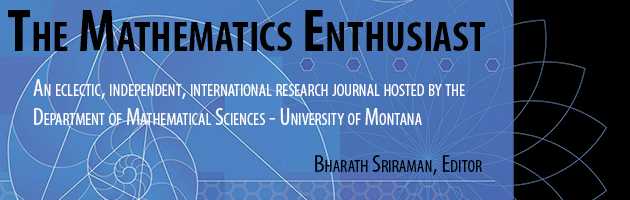•
•16

1-3

#### Abstract

Until the seventeenth century, rational numbers were represented as fractions. It was starting from that century, thanks to Simon Stevin, that for all practical purposes the use of decimal notation became widespread. Although decimal numbers are widely used, their organic development is lacking, while a vast literature propagates misconceptions about them, among both students and teachers. In particular, the case of the period 9 is especially interesting. In this paper, changing the point of view, we propose substituting the usual definitions of the periodic decimal representation of a rational number - the one obtained through long division introduced in the secondary school and the one obtained through the series concept for undergraduate level - with one which, instead of infinite progressions, uses a known property of fractions deducible from Euler's Totient Theorem. The long division becomes a convenient algorithm for obtaining the decimal expansion of a rational number. The new definition overcomes the difficulties noted in the literature. An elementary proof of Euler's Totient Theorem will be given depending only on Euclidean Division Theorem.

3

14

COinS